RS Aggarwal Class 6 Solutions Chapter 2 - Factors And Multiples

RS Aggarwal Class 6 Chapter 2 - Factors And Multiples Solutions Free PDF

Factors and Multiples are one of the most important topics for the students of class 6. In this chapter, the students will learn how to find factors of a given number, various types of numbers, general properties of divisibility, HCF, LCM, etc. To learn the methods or steps to find the factors and multiples students are advised to solve the RS Aggarwal Class 6 solutions Chapter 2 Factors and Multiples.

Students must practice these solutions to gain more marks in the exam. We have provided here all the exercise questions of RS Aggarwal Maths solution to help you to revise the complete syllabus. It is an important tool to prepare effectively for the Class 6 Maths examination. All the solutions are explained in a detailed step-by-step manner for the better understanding of the students. To prepare for exams, it is considered as one of the best reference material.

Download PDF of RS Aggarwal Class 6 Chapter 2- Factors and Multiples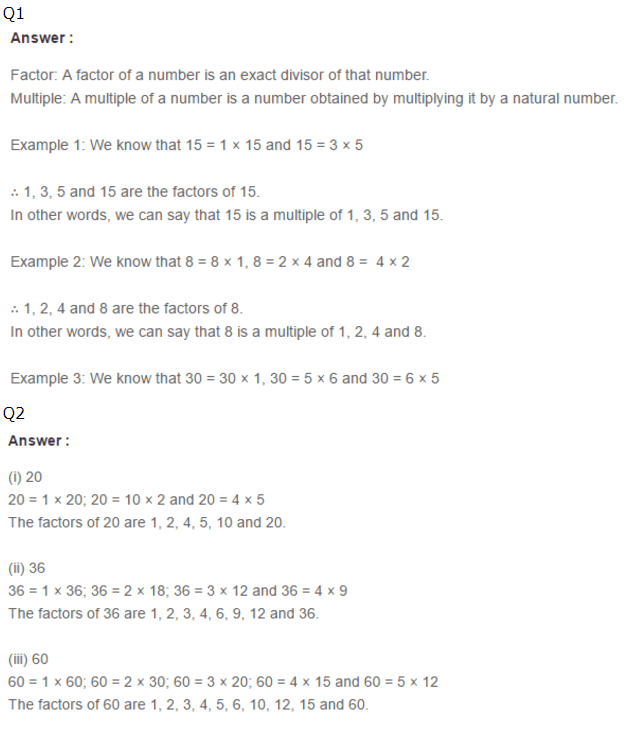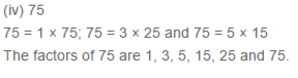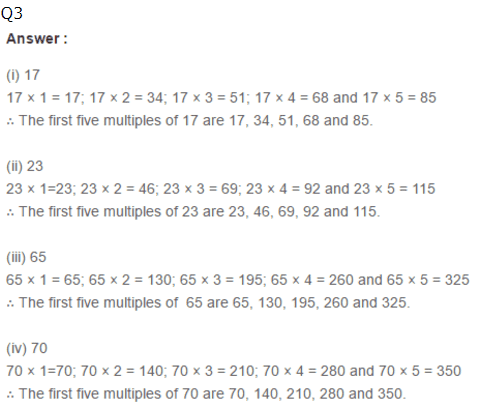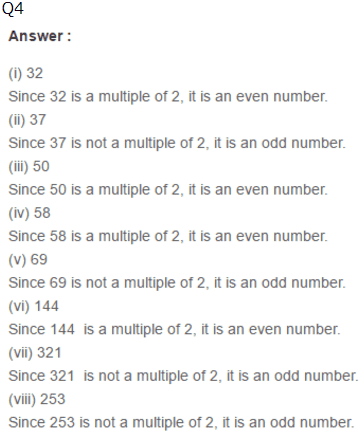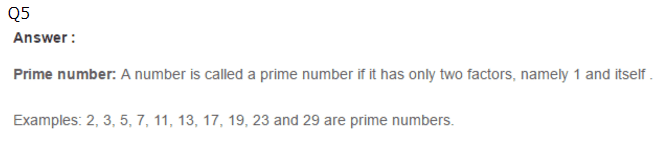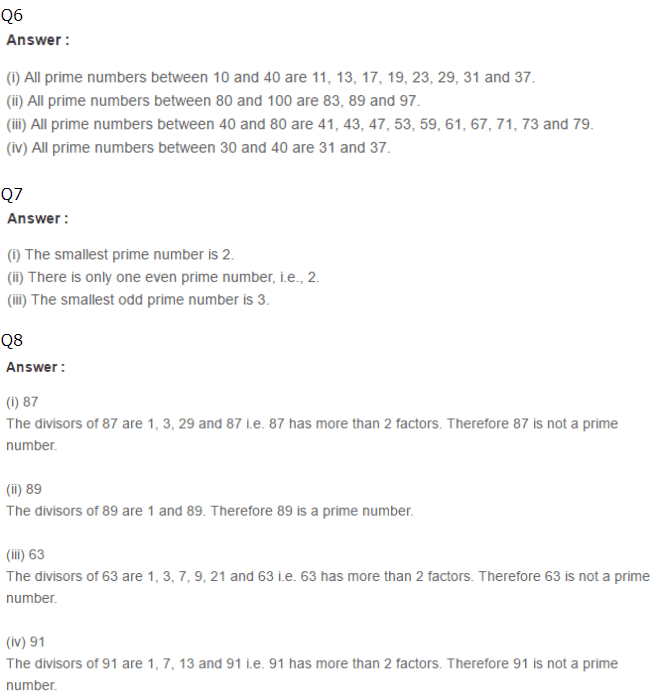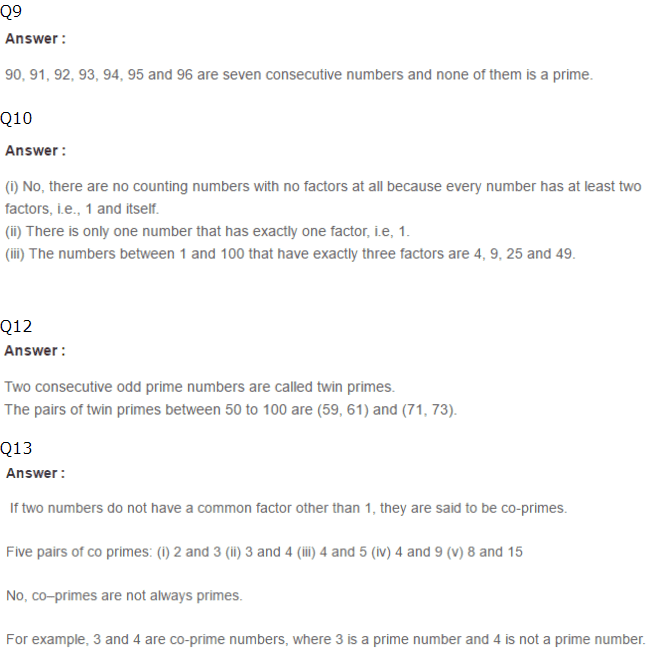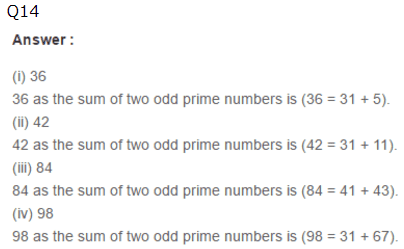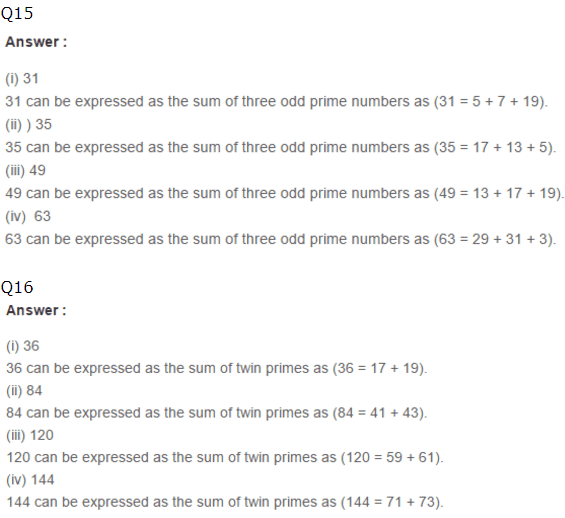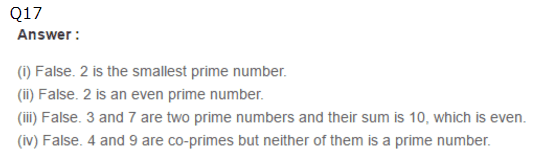RS Aggarwal Class 6 Solutions Chapter 2 – Factors and Multiples

Class 6 Chapter 2 solutions of RS Aggarwal provides all the answers in a proper stepwise method which makes you well versed with all the solutions including the tougher ones. If you want to score good marks in your Class 6 examination, then, practicing RS Aggarwal Class 6 Maths solutions is an utmost necessity. It is one of the best study material when it comes a providing a question bank to practice from and also test the students understanding of concepts.

Practise This Question

On a real number line, x1=4 and x2=14. What is the distance between these two points?